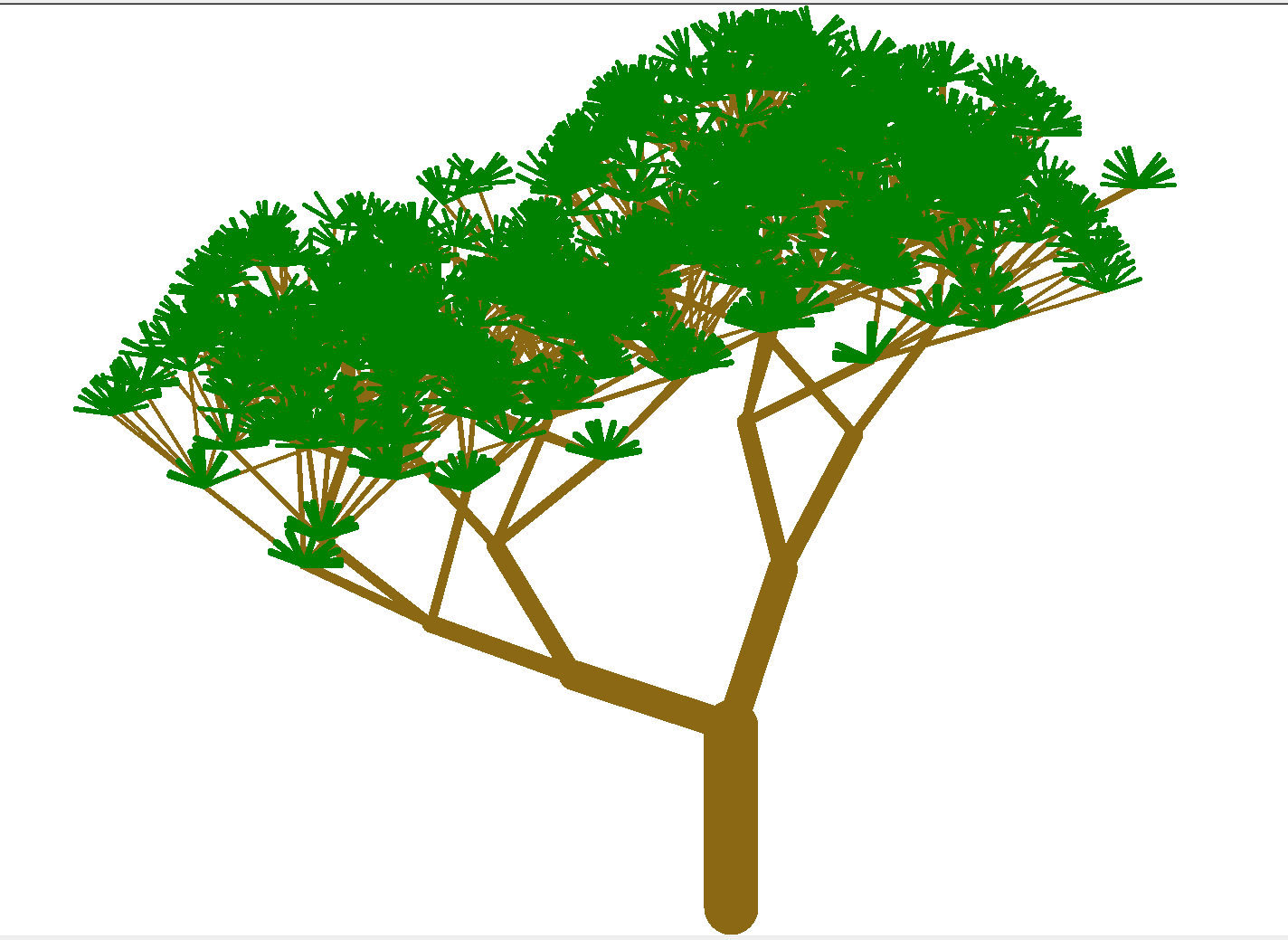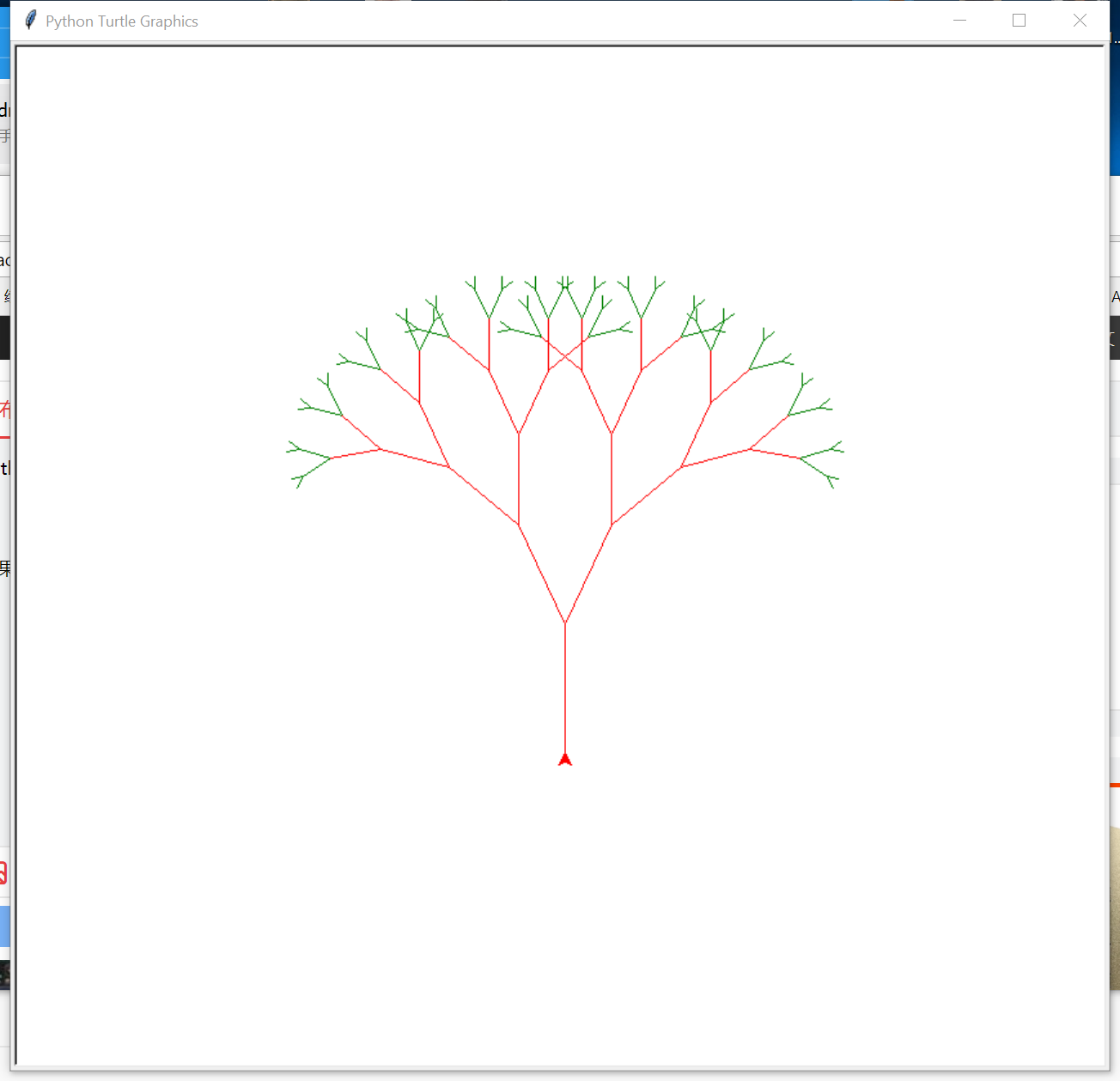• 主要介绍了Python+Turtle动态绘制一棵树实例分享，具有一定借鉴价值,需要的朋友可以参考下
• # 画一棵樱花 import turtle import random from turtle import * from time import sleep # 画樱花的躯干(60,t) def tree(branchLen,t): sleep(0.0005) if branchLen >3: if 8<= branchLen <=12: if ...
• #利用turtle模块画一棵树，包括枝干和树叶，并涂上颜色 import turtle import random turtle.screensize(10000,10000) turtle.Turtle().screen.delay(0) #取消延迟，有奇效 p=turtle.Pen() p.speed(0) #笔尖速度调至...
#利用turtle模块画一棵树，包括枝干和树叶，并涂上颜色
import turtle
import random
turtle.screensize(10000,10000)
turtle.Turtle().screen.delay(0)               #取消延迟，有奇效
p=turtle.Pen()
p.speed(0)                                    #笔尖速度调至最大
p.color('Goldenrod4')                         #树干颜色
p.seth(90)                                    #调整初始角度
pos=[[],[],[],[],[],[],[],[],[],[]]           #记录枝干节点位置
pos.append(p.pos())                        #将枝干节点位置存储到列表中
for i in range(6):                            #枝干层数
for pos_ in  pos[i]:
for j in range(round((3/2)**i)):      #每个枝干节点的枝干数
p.penup()                         #提笔并在枝干节点之间移动
p.goto(pos_)
p.pendown()
p.width(60*(4/7)**i)              #枝干宽度
p.forward(200*(11/12)**i)          #枝干长度
pos[i+1].append(p.pos())          #在列表中添加枝干接点
a=random.randint(-75,75)          #设置枝干方向
p.seth(a+90)
p.color('green')                              #叶子颜色
p.seth(90)
for i in range(4,7):
for pos_ in  pos[i]:
for j in range(10):      #每个枝干节点处的叶子数
p.penup()
p.goto(pos_)
p.pendown()
p.width(20*(4/5)**i)              #叶片宽度
p.forward(40)                     #叶片长度
a=random.randint(-90,90)          #叶片方向
p.seth(a+90)
turtle.mainloop()                             #显示绘图窗口
画出的树样例：展开全文python
• python3用turtle模块画一棵随机樱花 #!/usr/bin/env python # coding=utf-8 # 画一棵樱花 import turtle import random from turtle import * from time import sleep # 画樱花的躯干(60,t) def tree...
python3用turtle模块画一棵随机樱花树
#!/usr/bin/env python
# coding=utf-8
# 画一棵樱花

import turtle
import random
from turtle import *
from time import sleep

# 画樱花的躯干(60,t)
def tree(branchLen,t):
sleep(0.0005)
if branchLen >3:
if 8<= branchLen <=12:
if random.randint(0,2) == 0:
t.color('snow') # 白
else:
t.color('lightcoral') # 淡珊瑚色
t.pensize(branchLen / 3)
elif branchLen <8:
if random.randint(0,1) == 0:
t.color('snow')
else:
t.color('lightcoral') # 淡珊瑚色
t.pensize(branchLen / 2)
else:
t.color('sienna') # 赭(zhě)色
t.pensize(branchLen / 10) # 6
t.forward(branchLen)
a = 1.5 * random.random()
t.right(20*a)
b = 1.5 * random.random()
tree(
展开全文• 先说一下turtleturtle库是python的内部库，全称叫做海龟绘图(Turtle Graphics)，是在2.6版本后引入的个简单绘图工具，使用时直接导入即可。 #!\usr\bin\python #-*- coding:utf-8 -*- import turtle ...
先说一下turtle，turtle库是python的内部库，全称叫做海龟绘图(Turtle Graphics)，是在2.6版本后引入的一个简单绘图工具，使用时直接导入即可。

#!\usr\bin\python

#-*- coding:utf-8 -*-

import turtle

下面直接上代码：

#!\usr\bin\python

#-*- coding:utf-8 -*-

import turtle

def
draw_brach(
brach_length):

if brach_length >
5:

if brach_length <
40:

turtle.color(
'green')

else:

turtle.color(
'red')

#绘制右侧的树枝

turtle.forward(brach_length)

print(
'向前',brach_length)

turtle.right(
25)

print(
'右转20')

draw_brach(brach_length-
15)

#绘制左侧的树枝

turtle.left(
50)

print(
'右转40')

draw_brach(brach_length-
15)

if brach_length<
40:

turtle.color(
'green')

else:

turtle.color(
'red')

#返回之前的树枝上

turtle.right(
25)

print(
'右转20')

turtle.backward(brach_length)

print(
'返回',brach_length)

def
main():

turtle.left(
90)

turtle.penup()

turtle.backward(
150)

turtle.pendown()

turtle.color(
'red')

draw_brach(
100)

turtle.exitonclick()

if
__name__==
'__main__':

main()

运行效果：展开全文• # drawtree.py ...from turtle import Turtle, mainloop def tree(plist, l, a, f): """ plist is list of pens l is length of branch a is half of the angle between 2 branches f is...


# drawtree.py

from turtle import Turtle, mainloop

def tree(plist, l, a, f):
""" plist is list of pens
l is length of branch
a is half of the angle between 2 branches
f is factor by which branch is shortened
from level to level."""
if l > 5: #
lst = []
for p in plist:
p.forward(l)#沿着当前的方向画画Move the turtle forward by the specified distance, in the direction the turtle is headed.
q = p.clone()#Create and return a clone of the turtle with same position, heading and turtle properties.
p.left(a) #Turn turtle left by angle units
q.right(a)# turn turtle right by angle units, nits are by default degrees, but can be set via the degrees() and radians() functions.
lst.append(p)#将元素增加到列表的最后
lst.append(q)
tree(lst, l*f, a, f)

def main():
p = Turtle()
p.color("green")
p.pensize(5)
#p.setundobuffer(None)
p.hideturtle() #Make the turtle invisible. It’s a good idea to do this while you’re in the middle of doing some complex drawing,
#because hiding the turtle speeds up the drawing observably.
#p.speed(10)
# p.getscreen().tracer(1,0)#Return the TurtleScreen object the turtle is drawing on.
p.speed(10)
#TurtleScreen methods can then be called for that object.
p.left(90)# Turn turtle left by angle units. direction 调整画笔

p.penup() #Pull the pen up – no drawing when moving.
p.goto(0,-200)#Move turtle to an absolute position. If the pen is down, draw line. Do not change the turtle’s orientation.
p.pendown()# Pull the pen down – drawing when moving. 这三条语句是一个组合相当于先把笔收起来再移动到指定位置，再把笔放下开始画
#否则turtle一移动就会自动的把线画出来

#t = tree([p], 200, 65, 0.6375)
t = tree([p], 200, 65, 0.6375)

main()

http://www.jb51.net/article/116724.htm

转载于:https://www.cnblogs.com/wangsiting/p/7236327.html
展开全文• 关于工具介绍这里小生就不赘述了，这里附上个人觉得最详细的文档地址：https://docs.python.org/zh-cn/3/library/turtle.html?highlight=turtle#turtle.TurtleScreen先看效果图： 以上就是小生牺牲午休时间捣鼓...
• 最近翻到篇知乎，上面有不少用Python（大多是turtle库）绘制的树图，感觉很漂亮，我整理了一下，挑了一些我觉得不错的代码分享给大家（这些我都测试过，确实可以生成） one 樱花 动态生成樱花 效果图（这...python java js
• 最近翻到篇知乎，上面有不少用Python（大多是turtle库）绘制的树图，感觉很漂亮，我整理了一下，挑了一些我觉得不错的代码分享给大家（这些我都测试过，确实可以生成喔~） one 樱花 动态生成樱花 效果图（这个...python
• Turtle 最早来自于 LOGO 语言，是专门用于小孩子学习编程的，通过编程模拟turtle（海龟）在画板上爬行绘制图案，后来很多高级语言都移植了海龟绘图，python 从2.6之后也将 turtle 库加入了其内部库中。...
• 通常被定义为“个粗糙或零碎的几何形状，可以分成数个部分，且每部分都（至少近似地）是整体缩小后的形状”，即具有自相似的性质。分形（Fractal）词，是芒德勃罗创造出来的，其原意具有不规则、支离破碎等...python Fractal
• ## python教你画一棵树

千次阅读 2019-02-14 09:52:35
今天在知乎上找了个用 turtle 画树的 python 程序，起来看看。 其实这种程序，主要了解下大概思路即可，有兴趣的可以去熟悉下 turtle 中每个函数的使用方法和作用，我在玫瑰花那篇文章中也有过一些介绍...
• import turtle p = turtle.Turtle() turtle.colormode(255) p.speed(0) r = 0 g = 0 b = 0 length = 120 pen_size = 14 p.pensize(pen_size) p.penup() p.left(90) p.backward(length) p.pendown() p.forward(le.....python
• 用Python的turtle库绘图是很简单的,闲来无事就个玫瑰花,下面奉上源码…. 源码： ''' Created on Nov 18, 2017 @author: QiZhao ''' import turtle # 设置初始位置 turtle.penup() turtle.left(90) turtle.fd...
• Python用turtle模块画一棵漂亮的星星 非常漂亮的星星 每次执行都完全随机 五角星拥有随机颜色和大小 下面是源代码 import turtle , random turtle.screensize(900,600,'papayawhip') turtle.title('星星-羊')...python random
• import turtle as T import random import time # 樱花的躯干(60,t) def Tree(branch, t): time.sleep(0.0005) if branch > 3: if 8 <= branch <= 12: if random.randint(0, 2) =...python
• 不废话，上代码！！如何挂上自己女朋友名字？不会请留言 ... lv=turtle.Turtle() lv.hideturtle() lv.up() lv.goto(x,y)#定位到(x,y) def curvemove():#圆弧 for i in range(20): lv.right(10python
• 下面介绍使用Turtle库绘制一棵西兰花。 绘制一棵西兰花，从主干出发以一定的角度向左向右生成对称的枝干，再从每个枝干出发向左向右生成对称的枝干，循环此动作，并最终绘制出一棵漂亮的西兰花。 首先导入Turtle库，...
• 那么今天就一起来看看怎样在python中画一棵美丽的樱花～说到用python画画，那当然就是小乌龟Turtle库了，为啥是turtle呢，想象一个小乌龟，在一个横轴为x、纵轴为y的坐标系原点，(0,0)位置开始，它根据一组函数...
• 于是作为Python小白的我，也自己动手尝试了一下，画一颗很简单的，效果如下图： 效果自我感觉还行，如果想画的更好的画，可以自行添加树叶或者花朵，反正原理都基本一样，采用的都是递归的思想+随机原理，如有不...python
• 分享给大家一篇文章，教你怎样用Python画了一棵圣诞，快来学习。 如何用Python画一个圣诞呢？ 最简单： height = 5 ​ stars = 1 for i in range(height): print((' ' * (height - i)) + ('*' * stars)) ...
• import turtle def dfs(x,len): if x==2: turtle.pencolor('green') if x==5: return for i in range(2): if i==0: turtle.left(60) else: turtle.right(120) turtle.forward(len) dfs(x+1,le...
• 使用python的turtle画一棵好看的圣诞python 开发语言
• 虽然都不是会下子击垮自己的事情，但是积攒起来，还是会有突然感到疲惫和倦怠的时候，有种不知道下一步要走向哪里的无力感。 在这个城市留下了很多的回忆，但是身边的人个个离开，走到现在，似乎没有太多留恋...python
• % 函数 drawPineTree(x,y,h) % % 此函数用于绘制松树，树干位于 (x,y) 位置， 所需高度（h）的％。 它用于为图形提供比例。 %matlab...